# RD Sharma Solutions for Class 9 Maths Chapter 14 Quadrilaterals

## RD Sharma Solutions Class 9 Maths Chapter 14 – Free PDF Download

RD Sharma Solutions Class 9 Maths Chapter 14 Quadrilaterals consist of questions based on a polygon with 4 vertices or edges & 4 sides, which is termed as a quadrilateral. With 4 interior angles present, the sum of the interior angles of a quadrilateral is 360 degrees. Consider a quadrilateral PQRZ, with internal angles P, Q, R & Z. Then, ∠P + ∠Q + ∠R + ∠Z = 360o. For better grasping of given concepts, students are suggested to practice RD Sharma Solutions on a daily basis.

Basically, quadrilaterals are classified on the basis of their intersecting nature. If they are not intersecting they are called simple quadrilaterals. Otherwise, if they happen to self-intersect, it is a complex quadrilateral. Simple quadrilaterals are further classified into concave and convex quadrilaterals based on the position of diagonals and their interior angles. The RD Sharma Class 9 Solutions explain all the concepts from the chapter Quadrilaterals based on CBSE 2021-22 syllabus.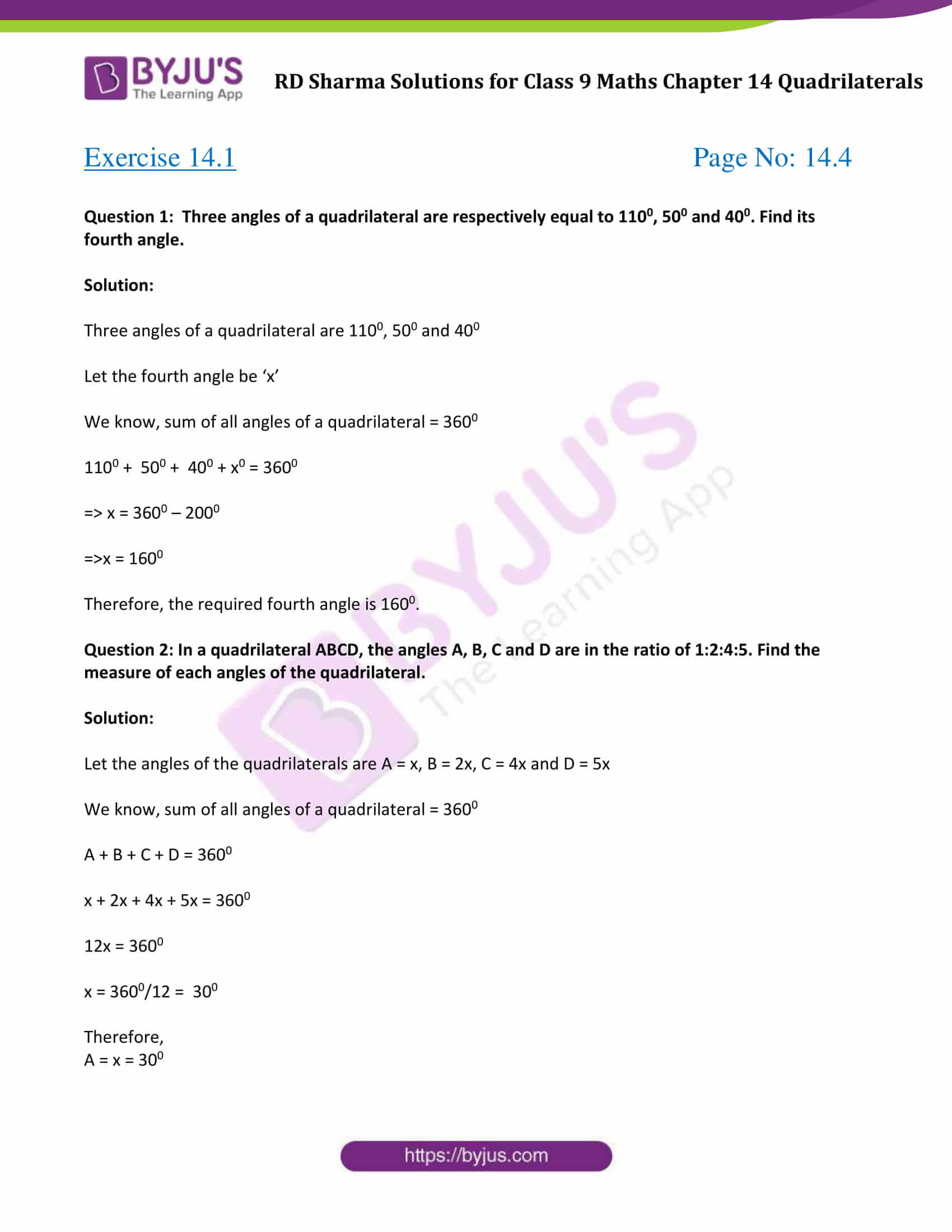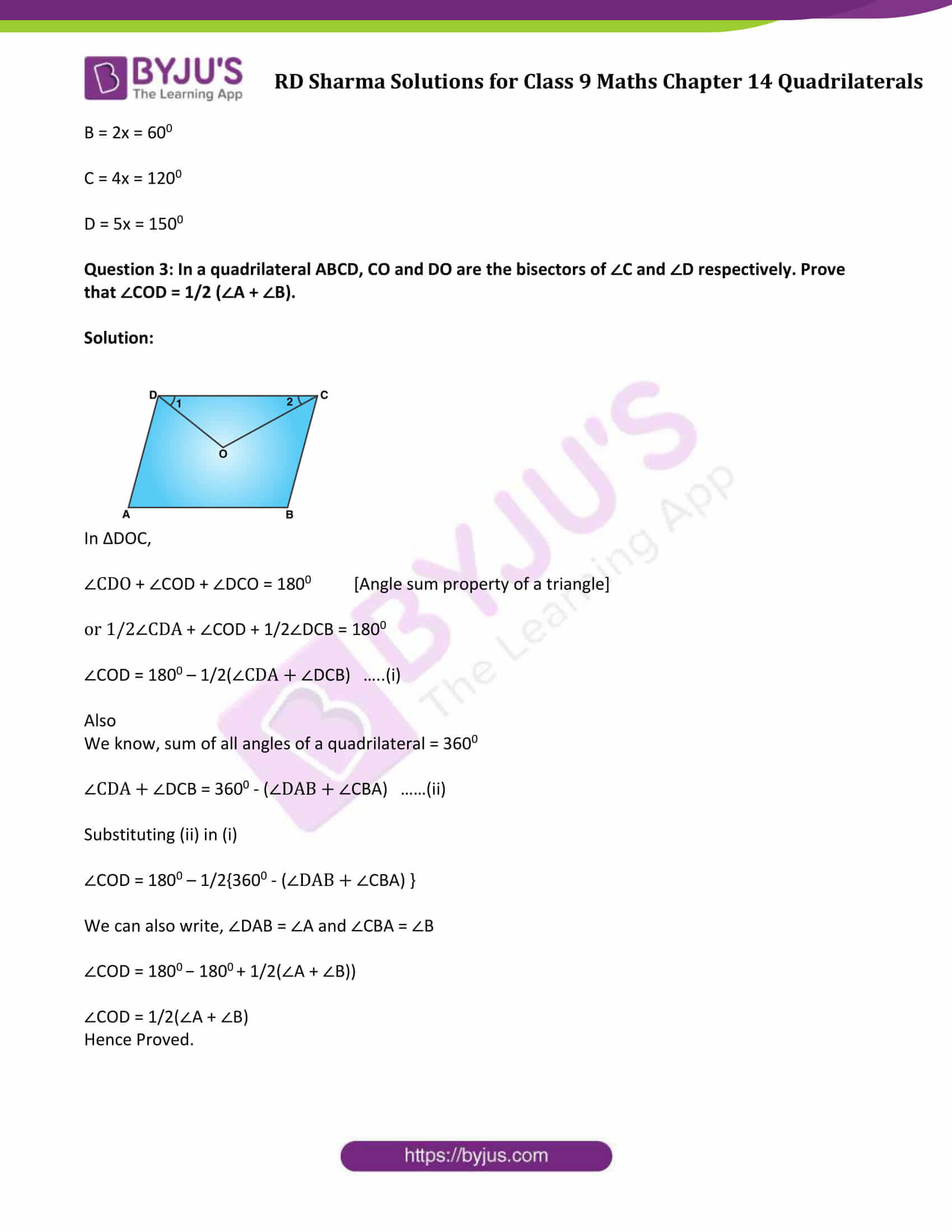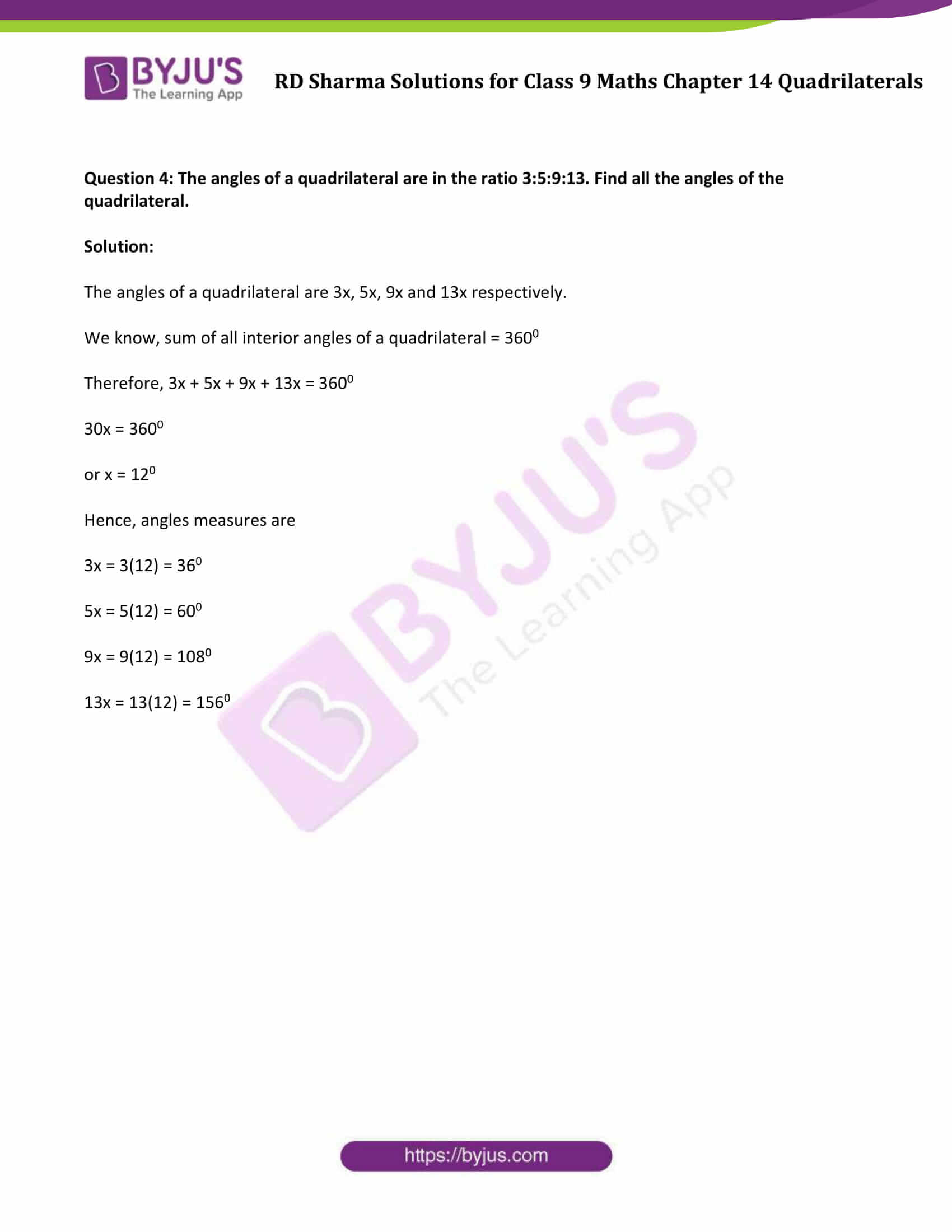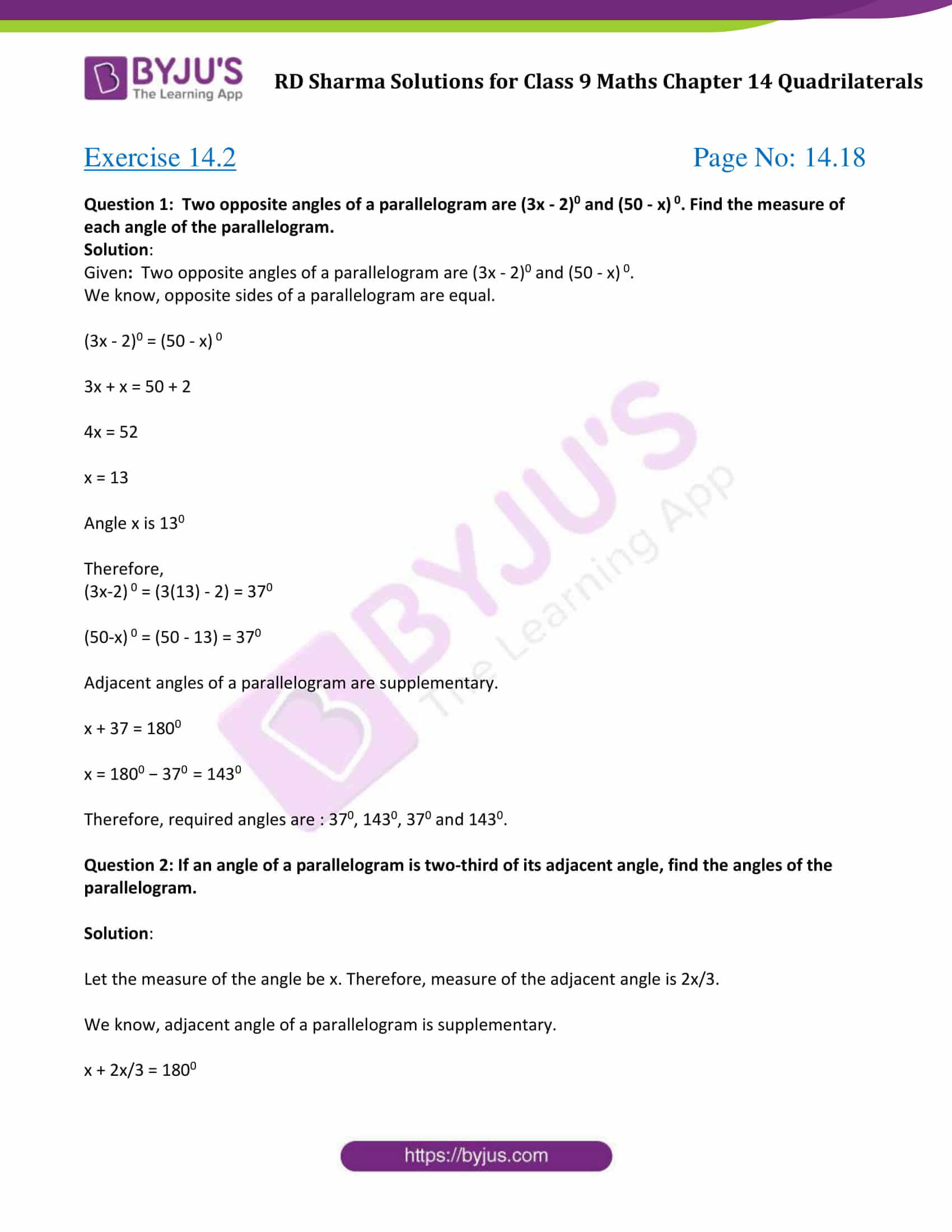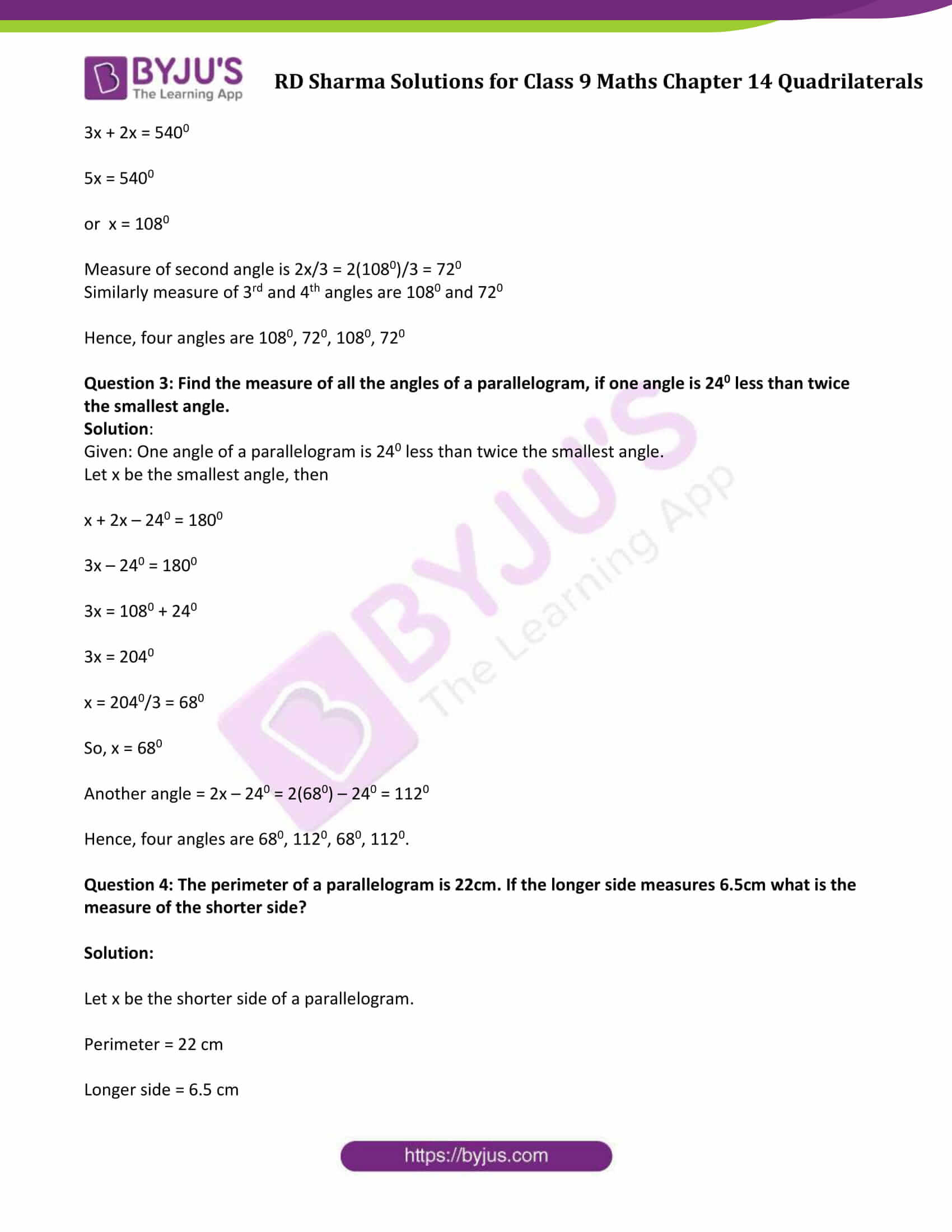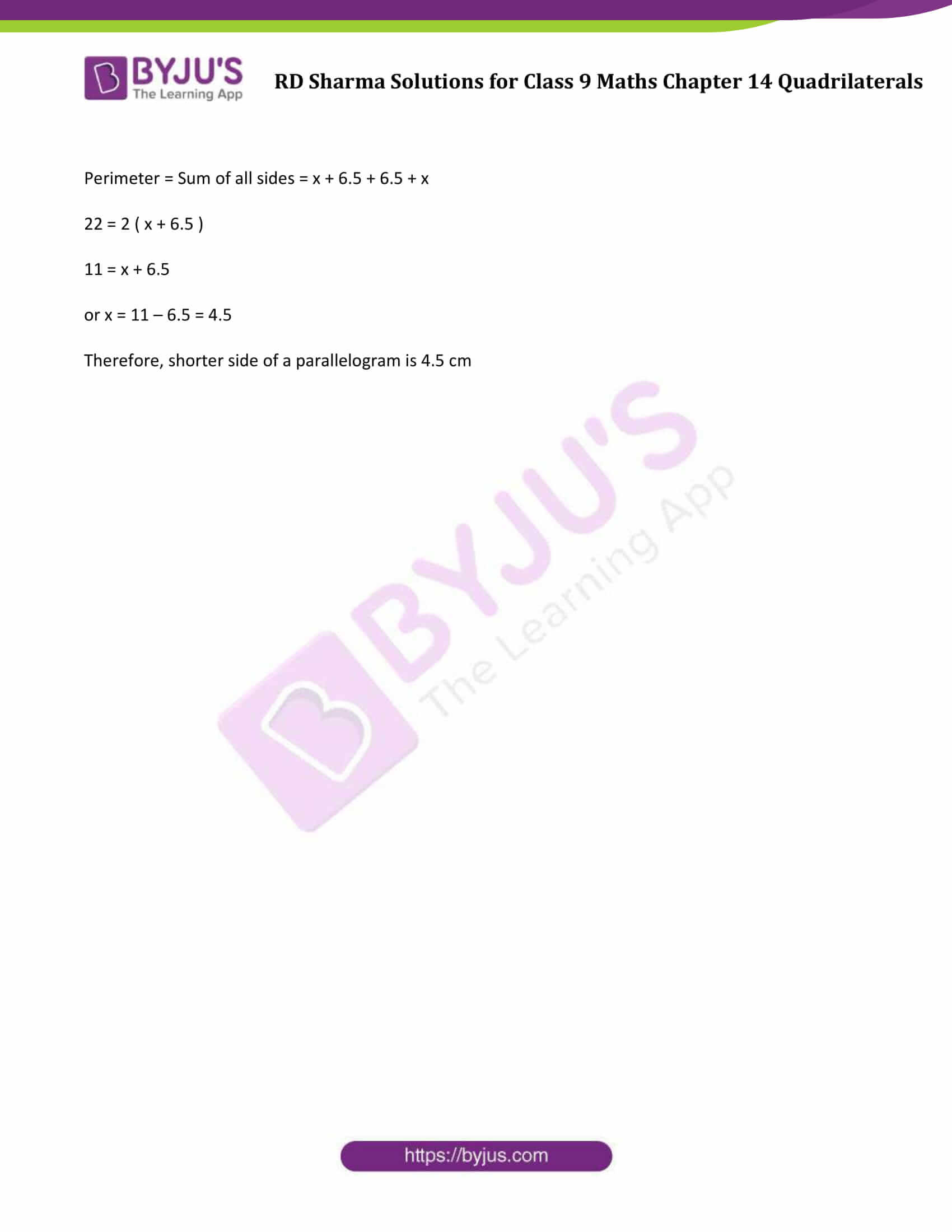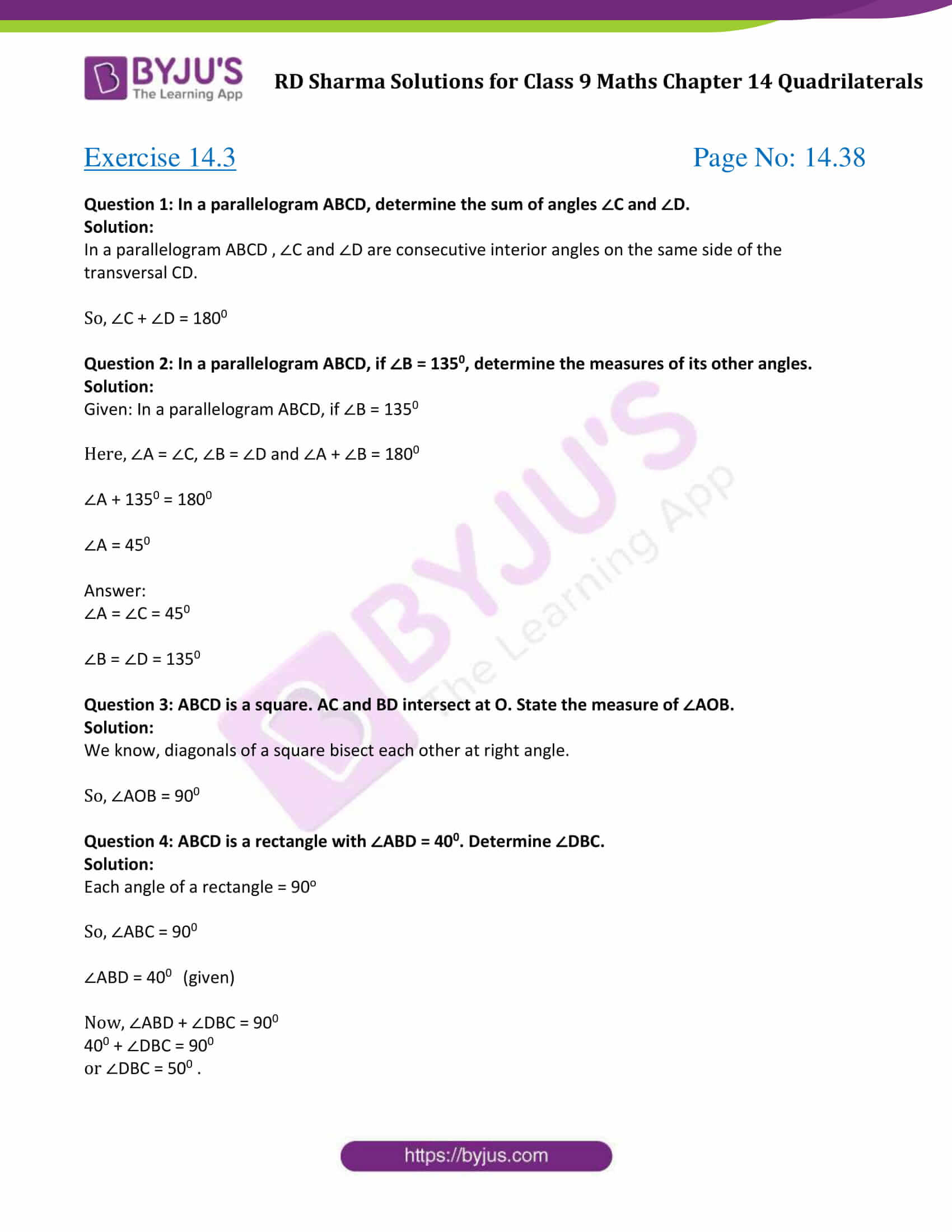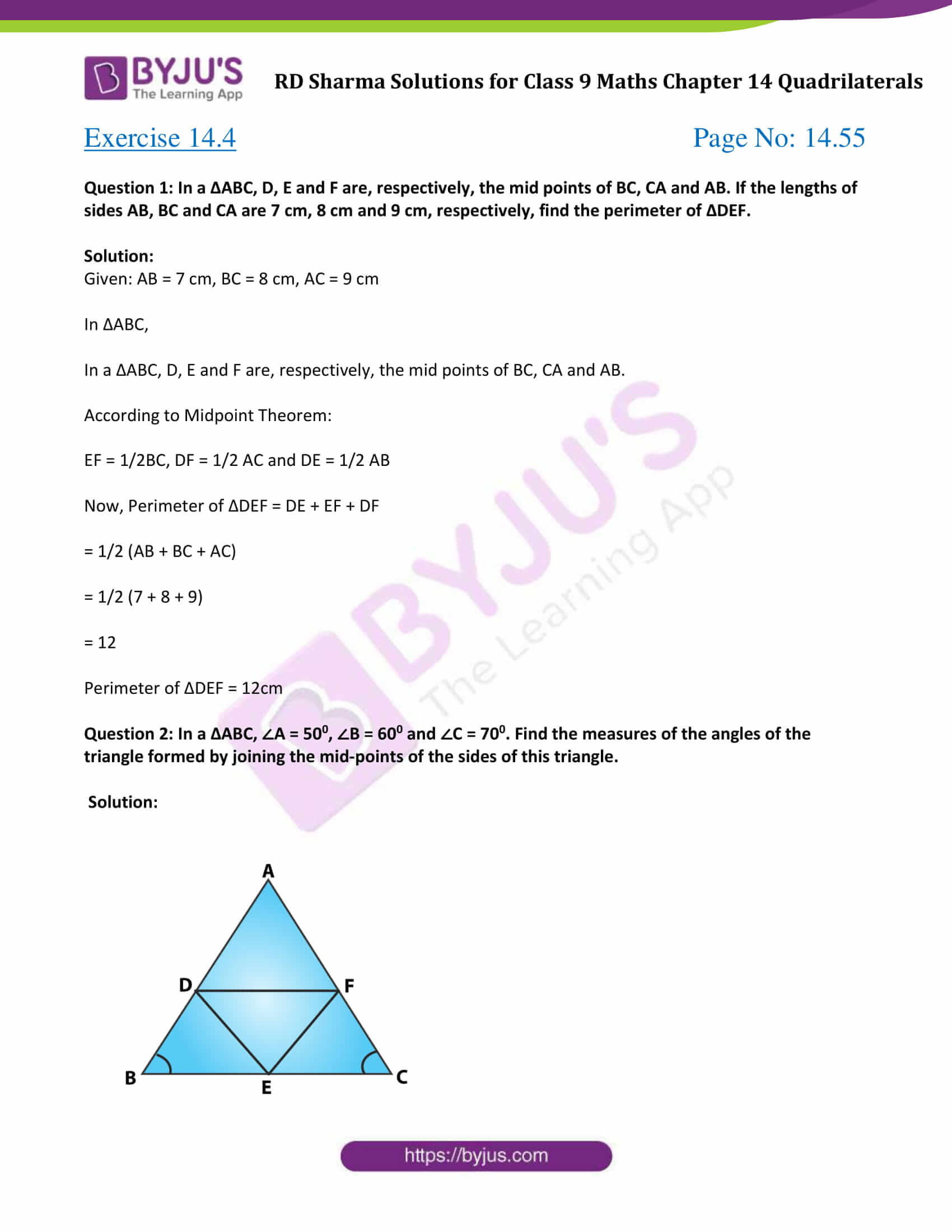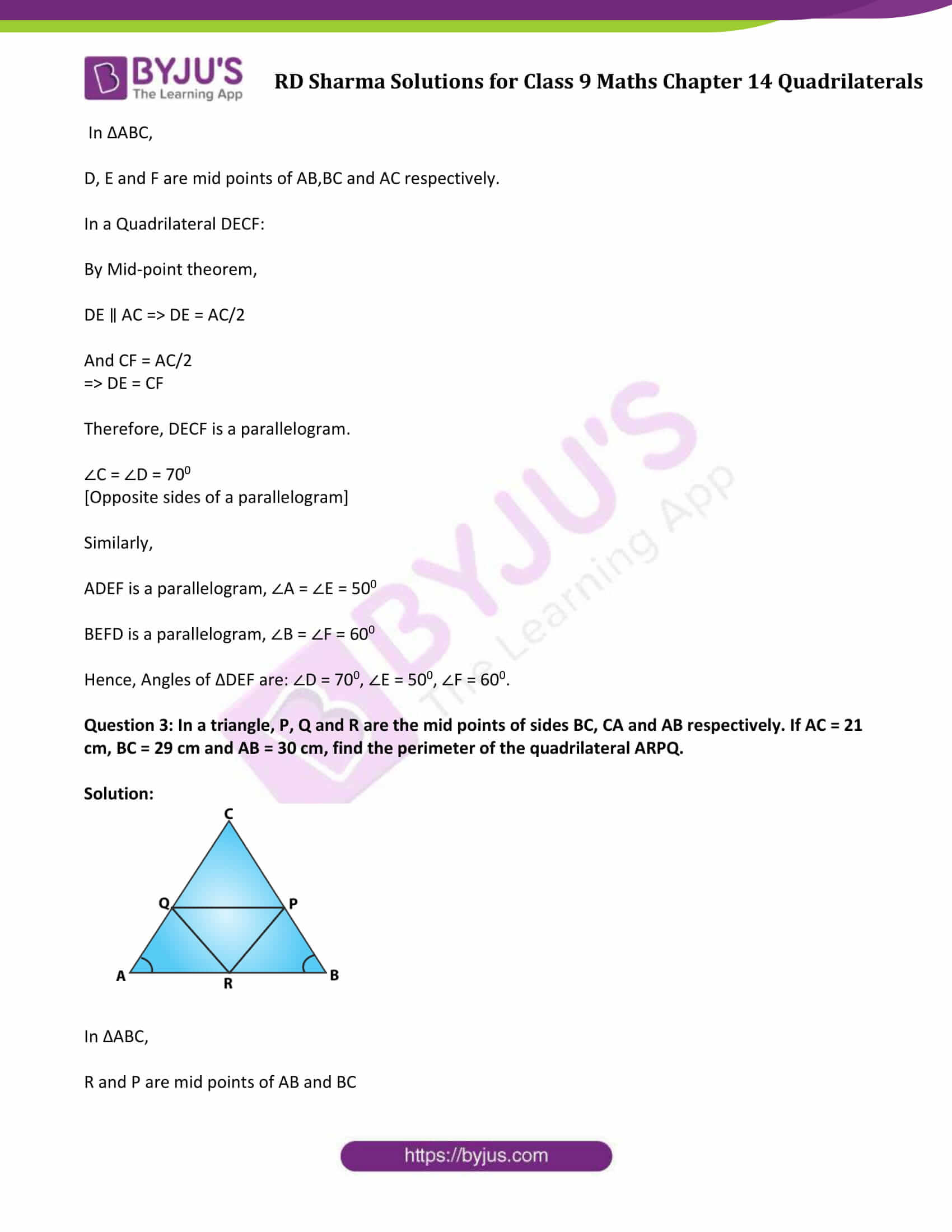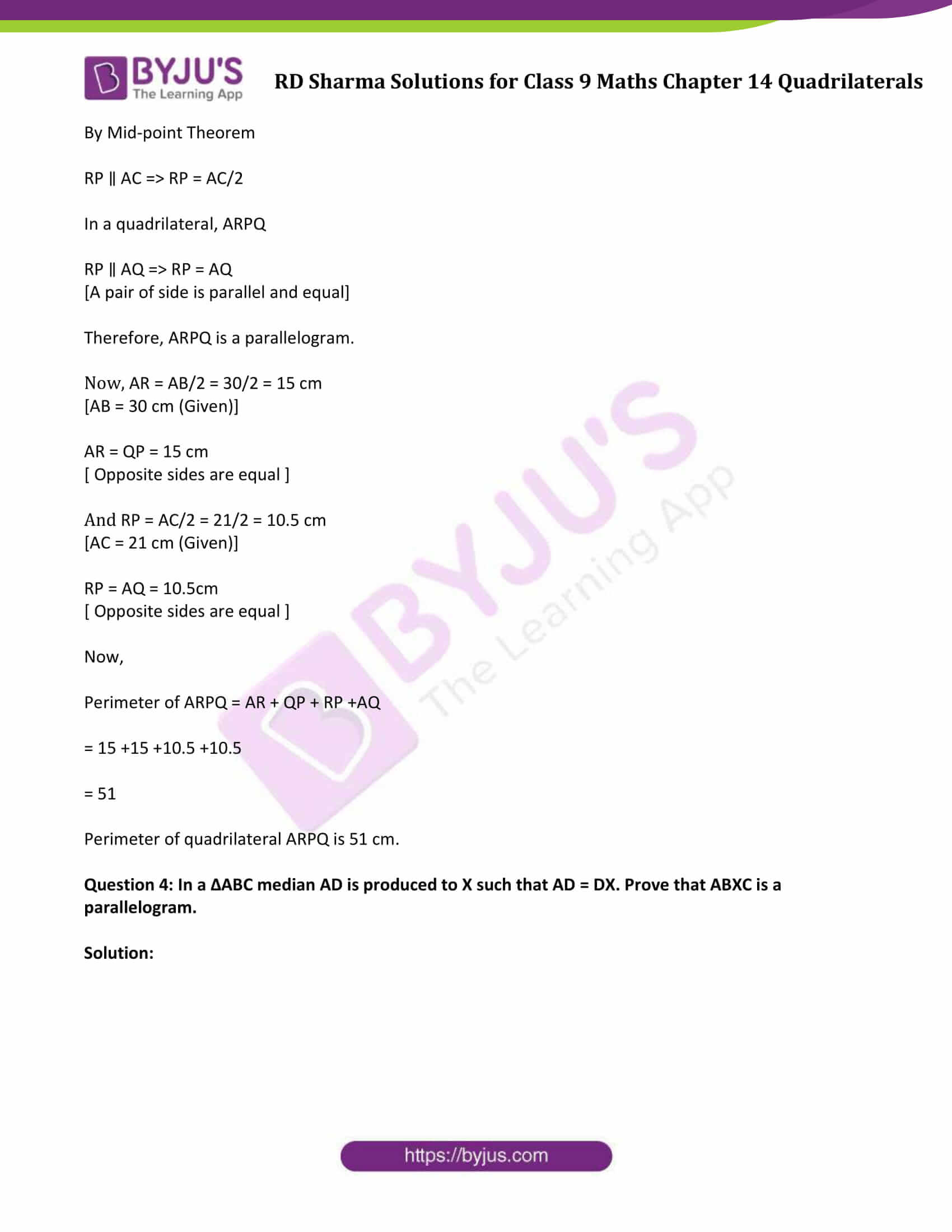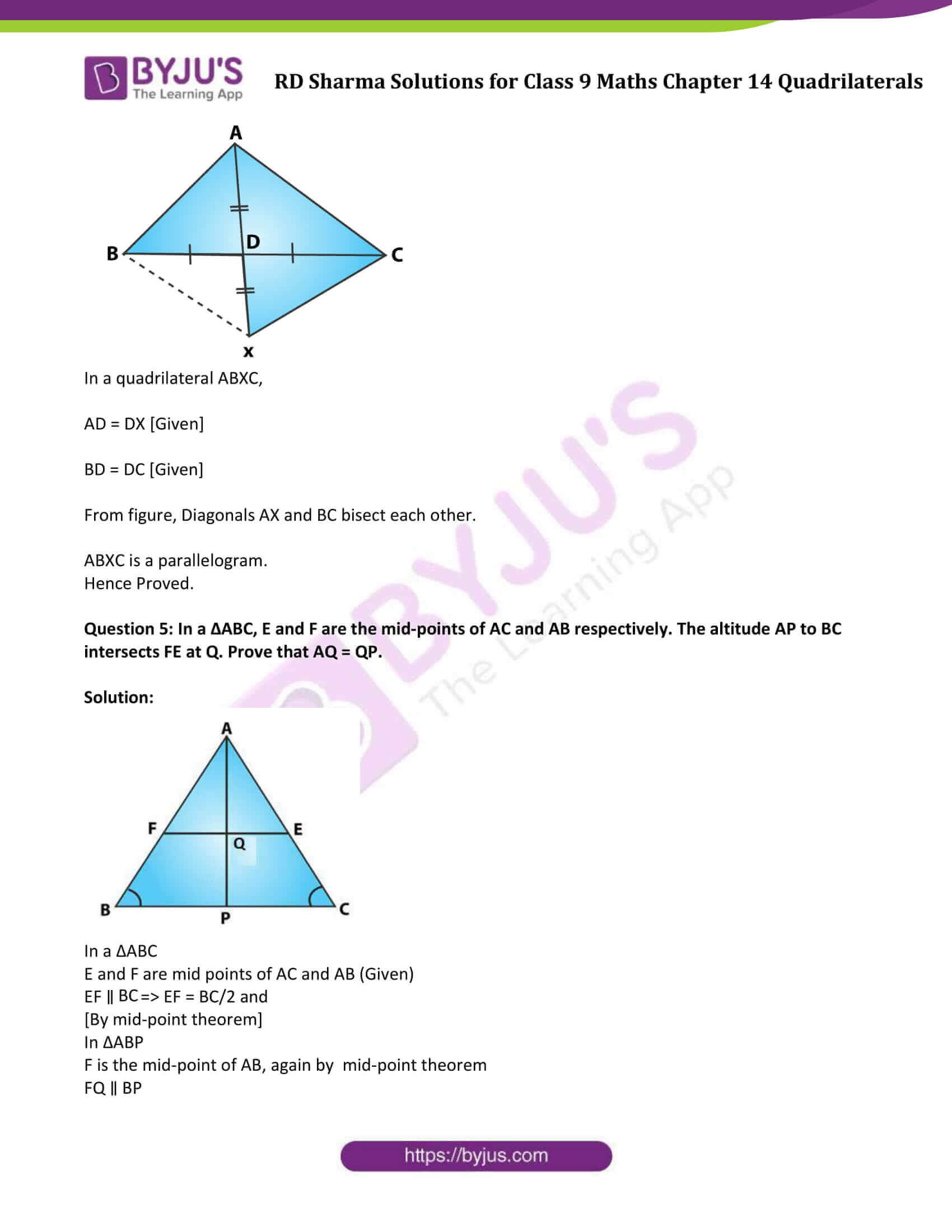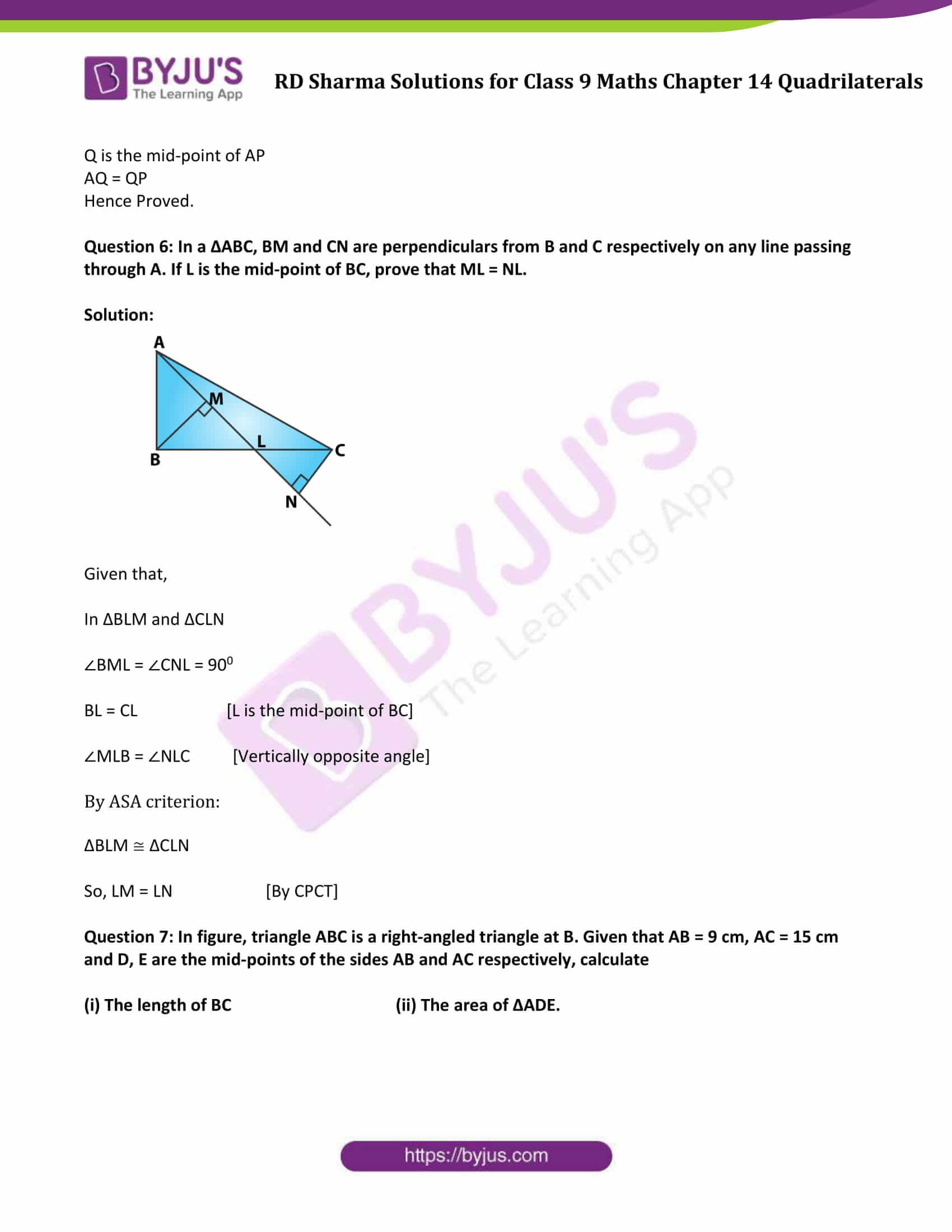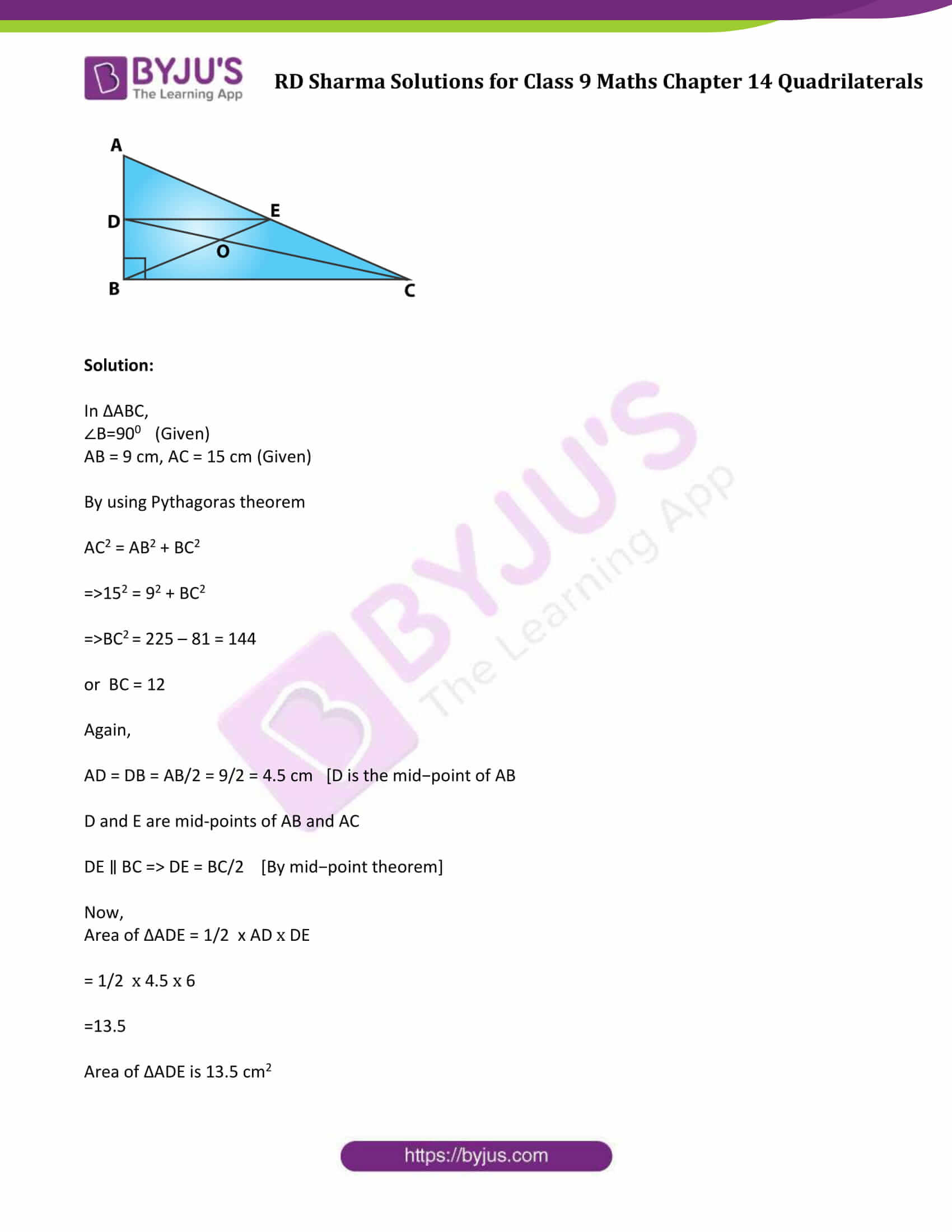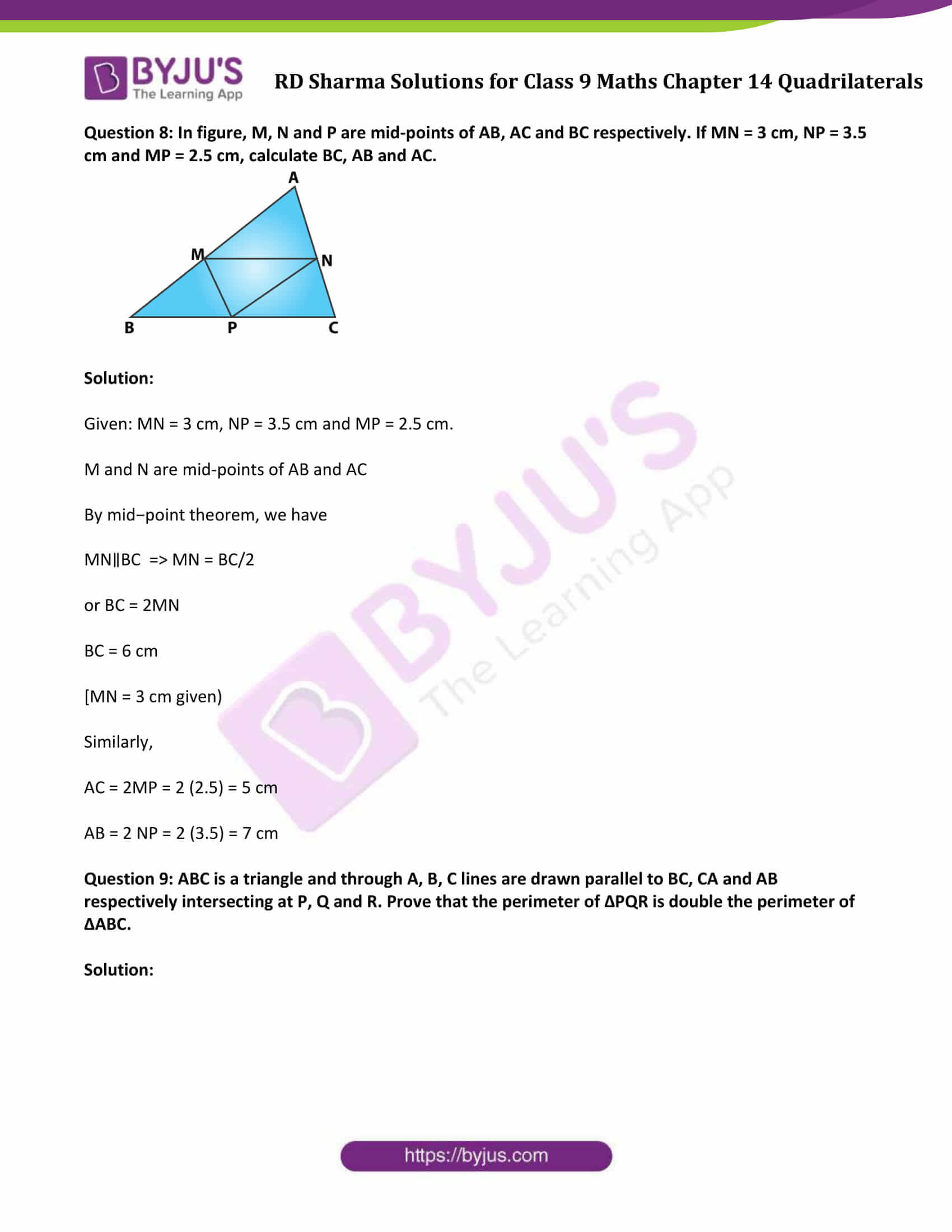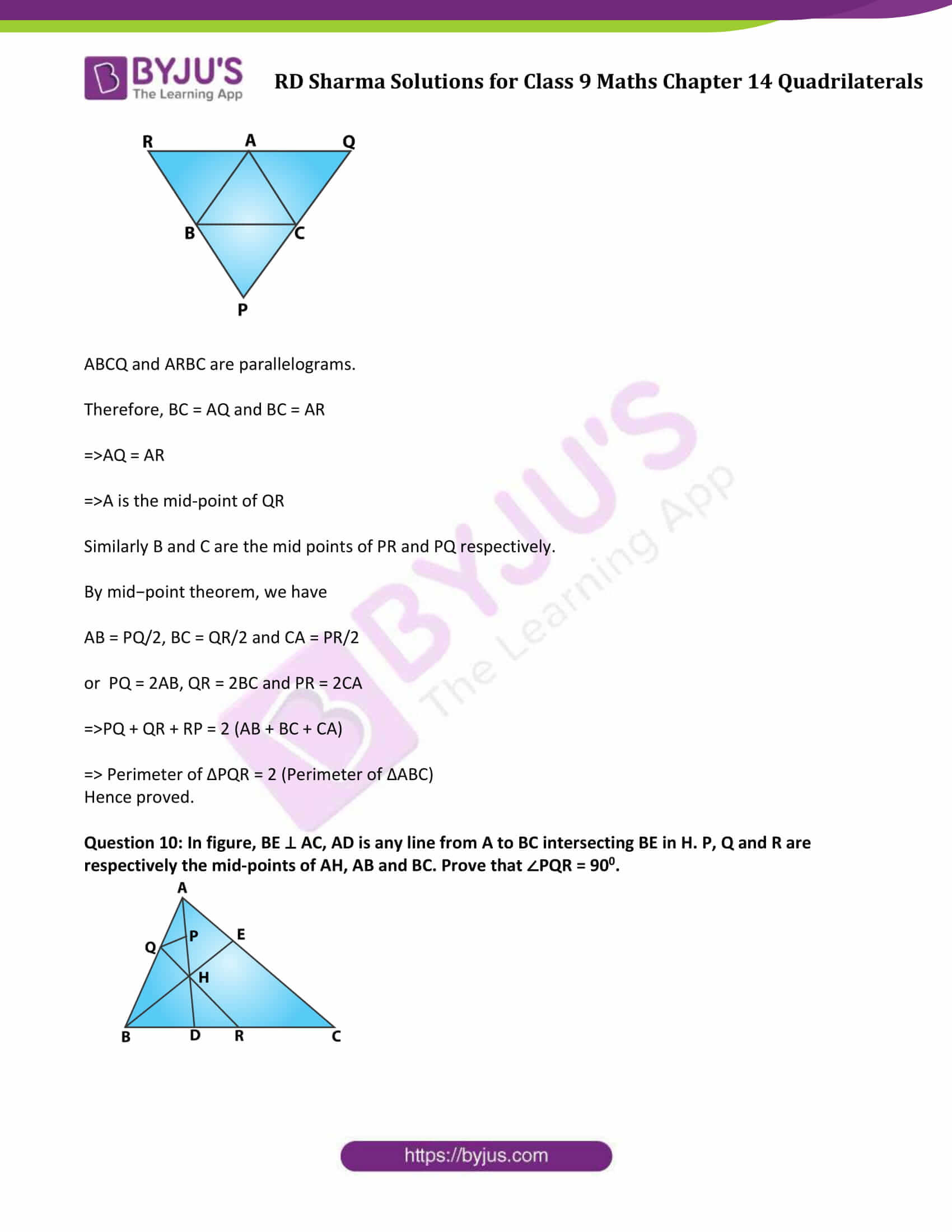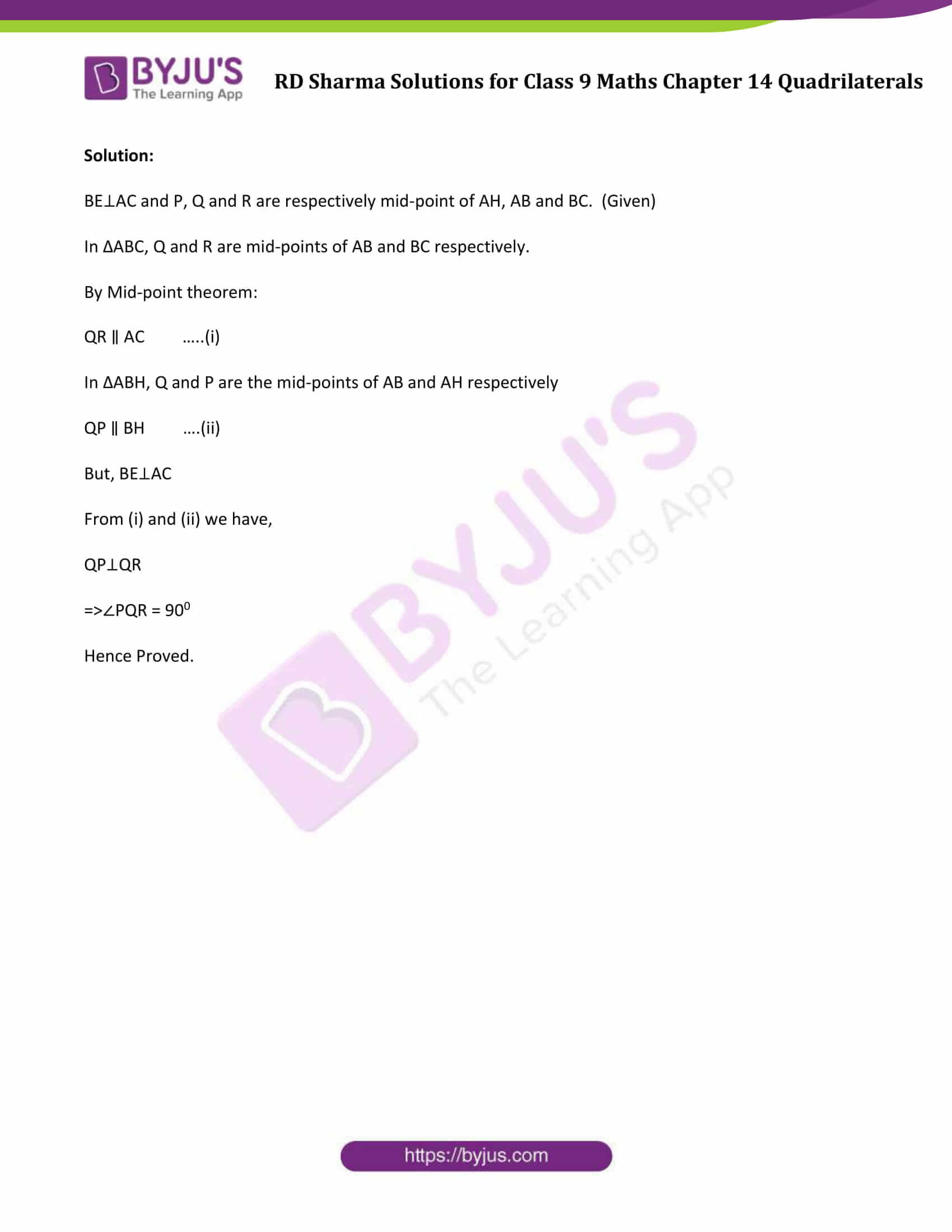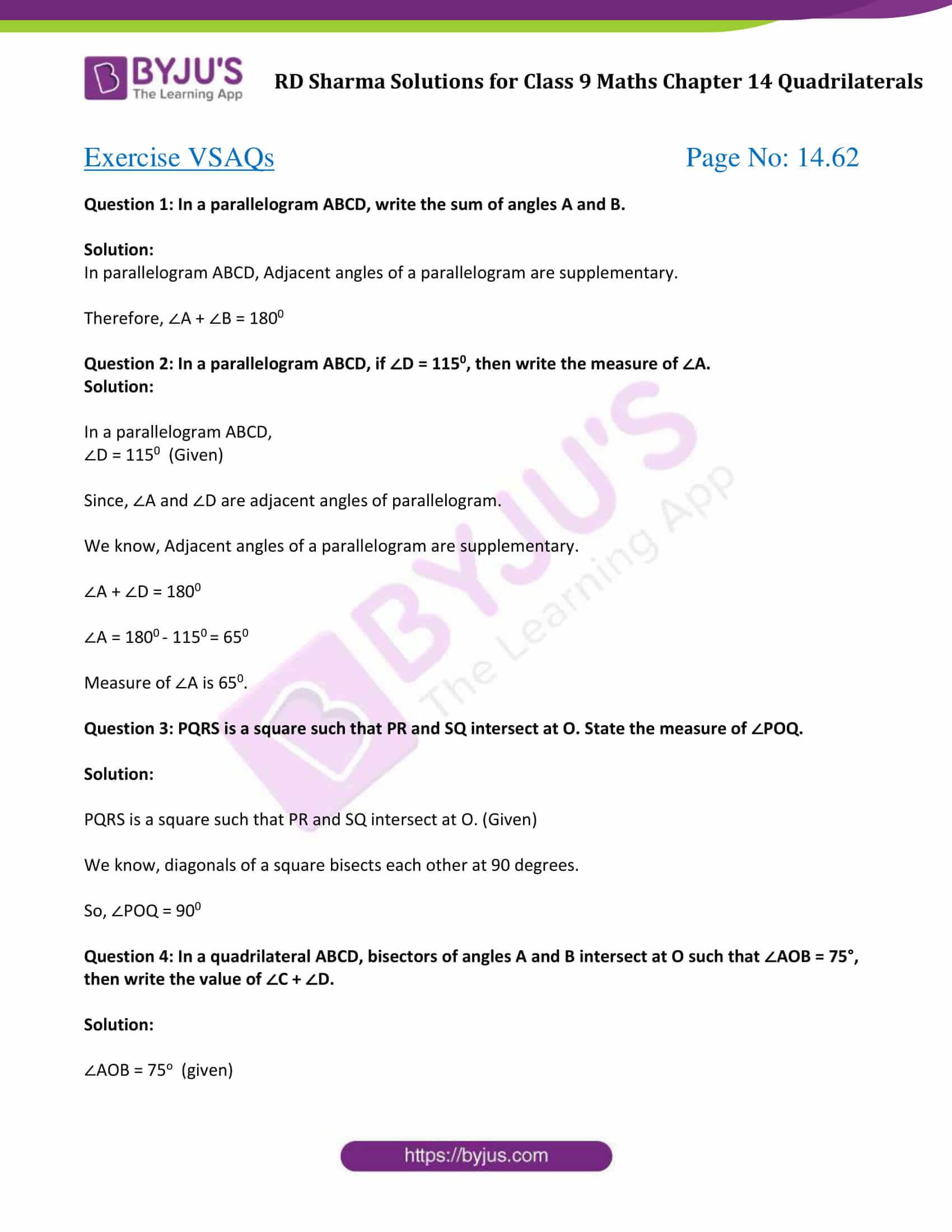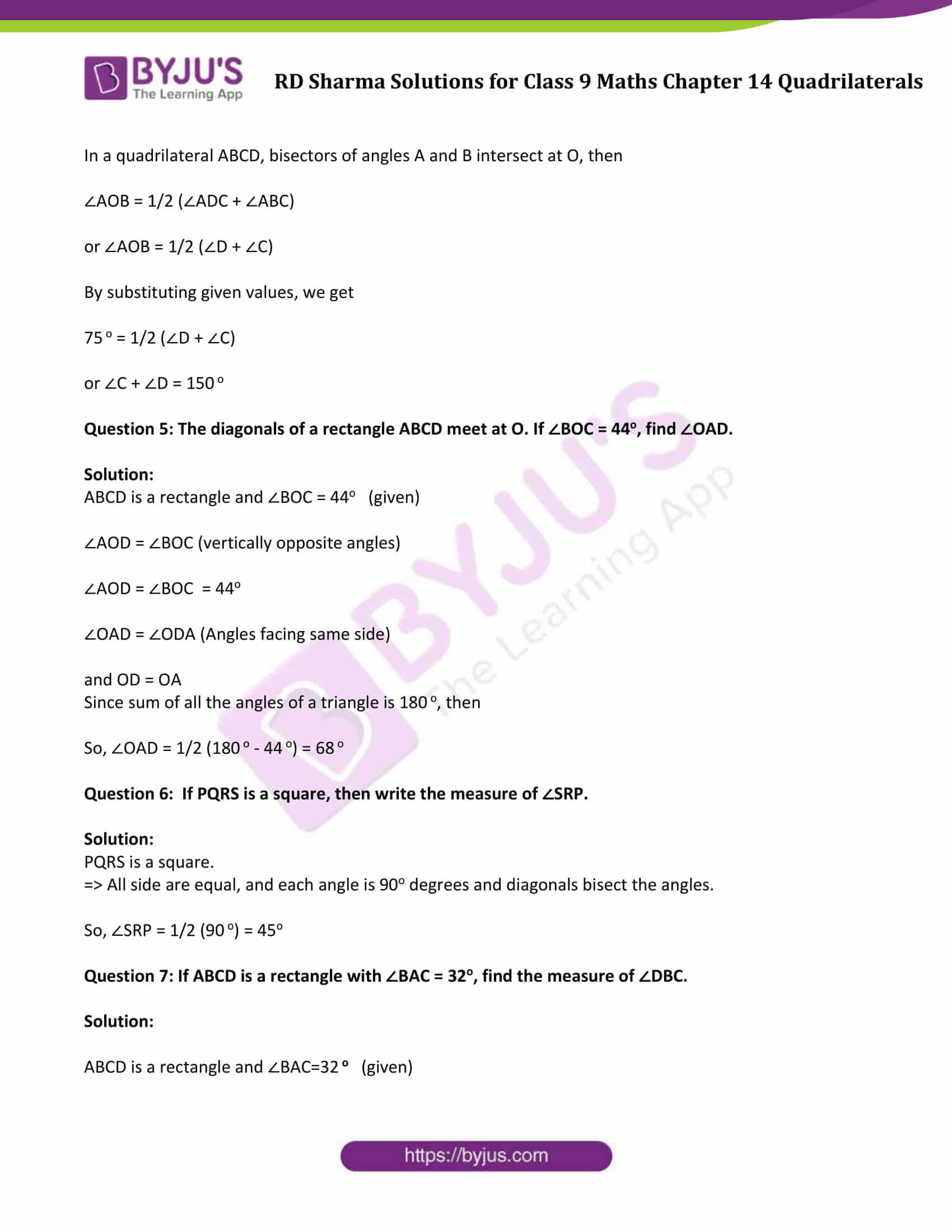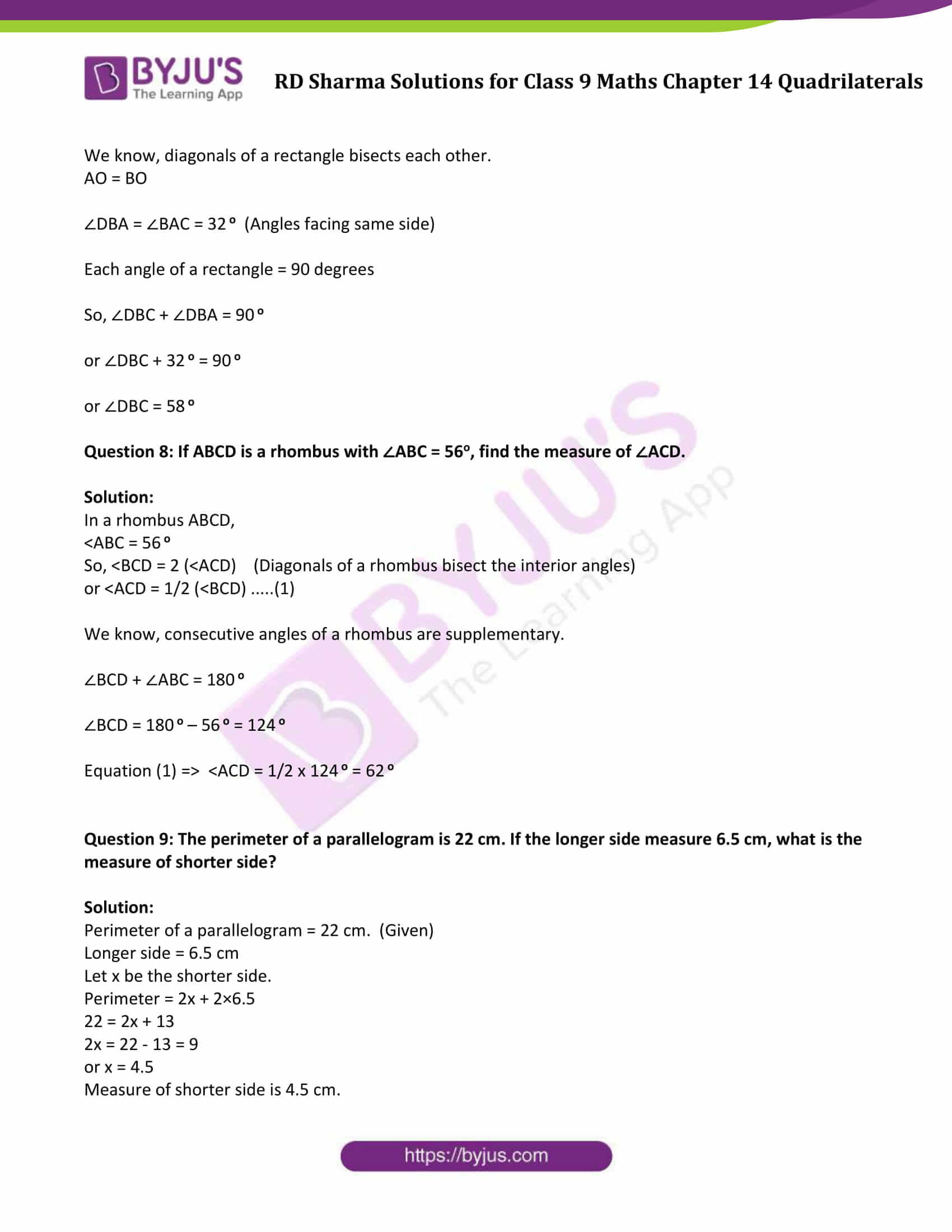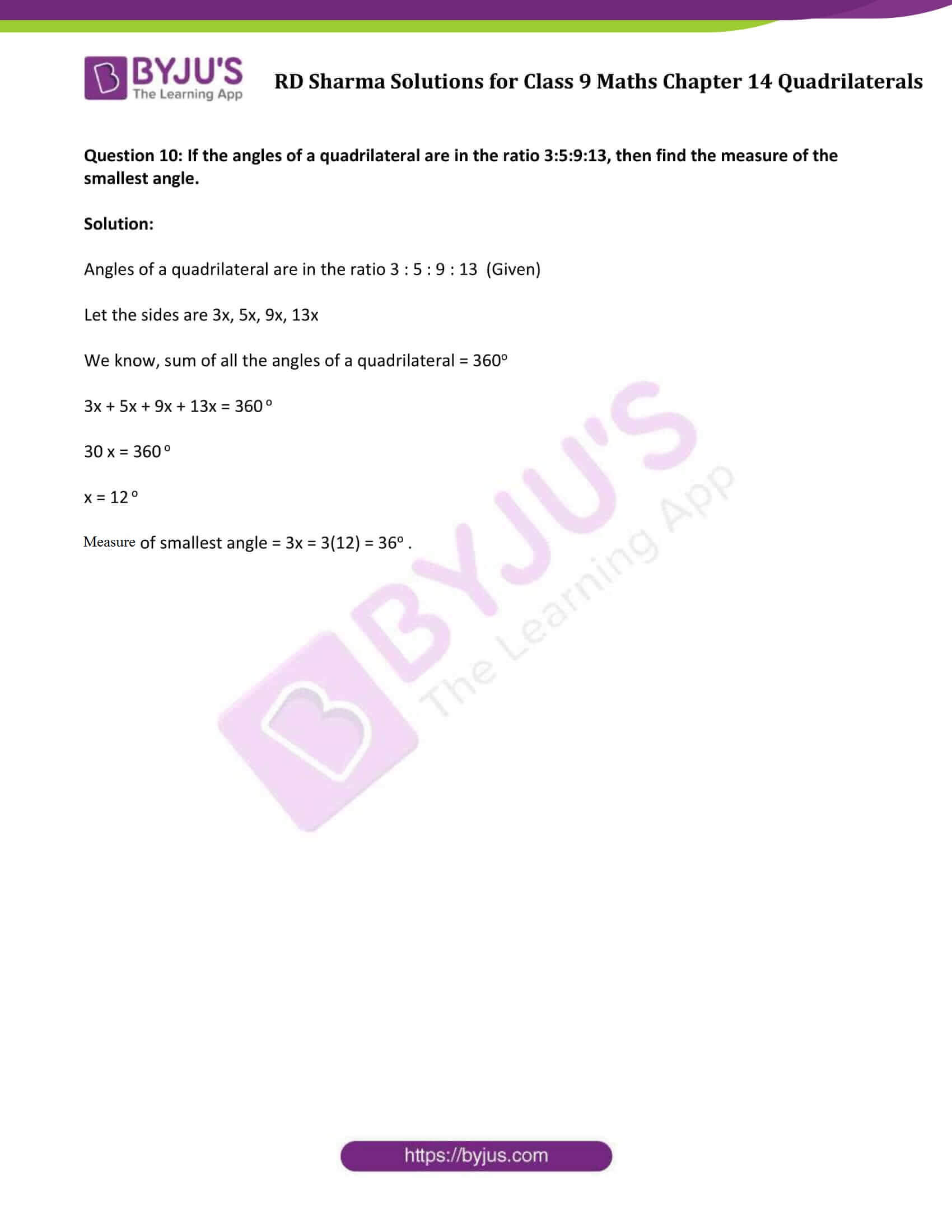### Exercise 14.1 Page No: 14.4

Question 1: Three angles of a quadrilateral are respectively equal to 1100, 500 and 400. Find its fourth angle.

Solution:

Three angles of a quadrilateral are 1100, 500 and 400

Let the fourth angle be ‘x’

We know, sum of all angles of a quadrilateral = 3600

1100 + 500 + 400 + x0 = 3600

⇒ x = 3600 – 2000

⇒x = 1600

Therefore, the required fourth angle is 1600.

Question 2: In a quadrilateral ABCD, the angles A, B, C and D are in the ratio of 1:2:4:5. Find the measure of each angles of the quadrilateral.

Solution:

Let the angles of the quadrilaterals are A = x, B = 2x, C = 4x and D = 5x

We know, sum of all angles of a quadrilateral = 3600

A + B + C + D = 3600

x + 2x + 4x + 5x = 3600

12x = 3600

x = 3600/12 = 300

Therefore,

A = x = 300

B = 2x = 600

C = 4x = 1200

D = 5x = 1500

Question 3: In a quadrilateral ABCD, CO and DO are the bisectors of ∠C and ∠D respectively. Prove that ∠COD = 1/2 (∠A + ∠B).

Solution: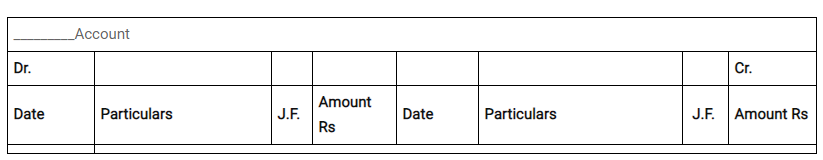In ΔDOC,

∠CDO + ∠COD + ∠DCO = 1800 [Angle sum property of a triangle]

or 1/2∠CDA + ∠COD + 1/2∠DCB = 1800

∠COD = 1800 – 1/2(∠CDA + ∠DCB) …..(i)

Also

We know, sum of all angles of a quadrilateral = 3600

∠CDA + ∠DCB = 3600 – (∠DAB + ∠CBA) ……(ii)

Substituting (ii) in (i)

∠COD = 1800 – 1/2{3600 – (∠DAB + ∠CBA) }

We can also write, ∠DAB = ∠A and ∠CBA = ∠B

∠COD = 1800 − 1800 + 1/2(∠A + ∠B))

∠COD = 1/2(∠A + ∠B)

Hence Proved.

Question 4: The angles of a quadrilateral are in the ratio 3:5:9:13. Find all the angles of the quadrilateral.

Solution:

The angles of a quadrilateral are 3x, 5x, 9x and 13x respectively.

We know, sum of all interior angles of a quadrilateral = 3600

Therefore, 3x + 5x + 9x + 13x = 3600

30x = 3600

or x = 120

Hence, angles measures are

3x = 3(12) = 360

5x = 5(12) = 600

9x = 9(12) = 1080

13x = 13(12) = 1560

### Exercise 14.2

Question 1: Two opposite angles of a parallelogram are (3x – 2)0 and (50 – x) 0. Find the measure of each angle of the parallelogram.

Solution:

Given: Two opposite angles of a parallelogram are (3x – 2)0 and (50 – x) 0.

We know, opposite sides of a parallelogram are equal.

(3x – 2)0 = (50 – x) 0

3x + x = 50 + 2

4x = 52

x = 13

Angle x is 130

Therefore,

(3x-2) 0 = (3(13) – 2) = 370

(50-x) 0 = (50 – 13) = 370

Adjacent angles of a parallelogram are supplementary.

x + 37 = 1800

x = 1800 − 370 = 1430

Therefore, required angles are : 370, 1430, 370 and 1430.

Question 2: If an angle of a parallelogram is two-third of its adjacent angle, find the angles of the parallelogram.

Solution:

Let the measure of the angle be x. Therefore, measure of the adjacent angle is 2x/3.

We know, adjacent angle of a parallelogram is supplementary.

x + 2x/3 = 1800

3x + 2x = 5400

5x = 5400

or x = 1080

Measure of second angle is 2x/3 = 2(1080)/3 = 720

Similarly measure of 3rd and 4th angles are 1080 and 720

Hence, four angles are 1080, 720, 1080, 720

Question 3: Find the measure of all the angles of a parallelogram, if one angle is 240 less than twice the smallest angle.

Solution:

Given: One angle of a parallelogram is 240 less than twice the smallest angle.

Let x be the smallest angle, then

x + 2x – 240 = 1800

3x – 240 = 1800

3x = 1080 + 240

3x = 2040

x = 2040/3 = 680

So, x = 680

Another angle = 2x – 240 = 2(680) – 240 = 1120

Hence, four angles are 680, 1120, 680, 1120.

Question 4: The perimeter of a parallelogram is 22cm. If the longer side measures 6.5cm what is the measure of the shorter side?

Solution:

Let x be the shorter side of a parallelogram.

Perimeter = 22 cm

Longer side = 6.5 cm

Perimeter = Sum of all sides = x + 6.5 + 6.5 + x

22 = 2 ( x + 6.5 )

11 = x + 6.5

or x = 11 – 6.5 = 4.5

Therefore, shorter side of a parallelogram is 4.5 cm

### Exercise 14.3

Question 1: In a parallelogram ABCD, determine the sum of angles ∠C and ∠D.

Solution:

In a parallelogram ABCD , ∠C and ∠D are consecutive interior angles on the same side of the transversal CD.

So, ∠C + ∠D = 1800

Question 2: In a parallelogram ABCD, if ∠B = 1350, determine the measures of its other angles.

Solution:

Given: In a parallelogram ABCD, if ∠B = 1350

Here, ∠A = ∠C, ∠B = ∠D and ∠A + ∠B = 1800

∠A + 1350 = 1800

∠A = 450

∠A = ∠C = 450

∠B = ∠D = 1350

Question 3: ABCD is a square. AC and BD intersect at O. State the measure of ∠AOB.

Solution:

We know, diagonals of a square bisect each other at right angle.

So, ∠AOB = 900

Question 4: ABCD is a rectangle with ∠ABD = 400. Determine ∠DBC.

Solution:

Each angle of a rectangle = 90o

So, ∠ABC = 900

∠ABD = 400 (given)

Now, ∠ABD + ∠DBC = 900

400 + ∠DBC = 900

or ∠DBC = 500 .

### Exercise 14.4

Question 1: In a ΔABC, D, E and F are, respectively, the mid points of BC, CA and AB. If the lengths of sides AB, BC and CA are 7 cm, 8 cm and 9 cm, respectively, find the perimeter of ΔDEF.

Solution:

Given: AB = 7 cm, BC = 8 cm, AC = 9 cm

In ∆ABC,

In a ΔABC, D, E and F are, respectively, the mid points of BC, CA and AB.

According to Midpoint Theorem:

EF = 1/2BC, DF = 1/2 AC and DE = 1/2 AB

Now, Perimeter of ∆DEF = DE + EF + DF

= 1/2 (AB + BC + AC)

= 1/2 (7 + 8 + 9)

= 12

Perimeter of ΔDEF = 12cm

Question 2: In a ΔABC, ∠A = 500, ∠B = 600 and ∠C = 700. Find the measures of the angles of the triangle formed by joining the mid-points of the sides of this triangle.

Solution: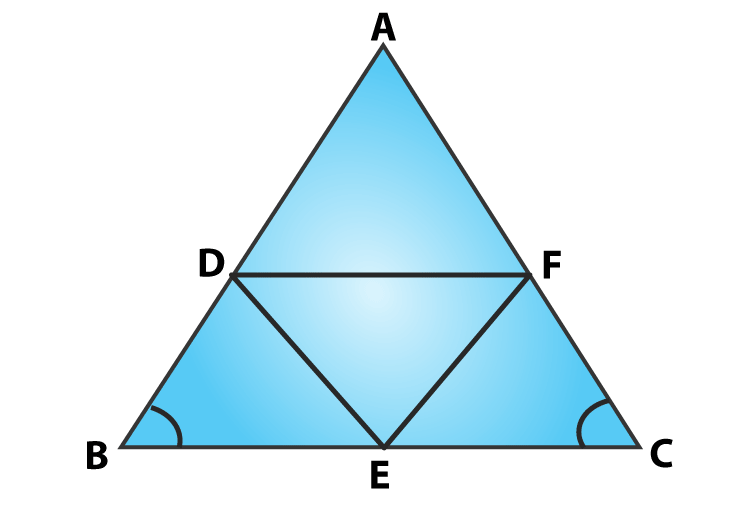In ΔABC,

D, E and F are mid points of AB,BC and AC respectively.

By Mid-point theorem,

DE ∥ AC ⇒ DE = AC/2

And CF = AC/2

⇒ DE = CF

Therefore, DECF is a parallelogram.

∠C = ∠D = 700

[Opposite sides of a parallelogram]

Similarly,

ADEF is a parallelogram, ∠A = ∠E = 500

BEFD is a parallelogram, ∠B = ∠F = 600

Hence, Angles of ΔDEF are: ∠D = 700, ∠E = 500, ∠F = 600.

Question 3: In a triangle, P, Q and R are the mid points of sides BC, CA and AB respectively. If AC = 21 cm, BC = 29 cm and AB = 30 cm, find the perimeter of the quadrilateral ARPQ.

Solution: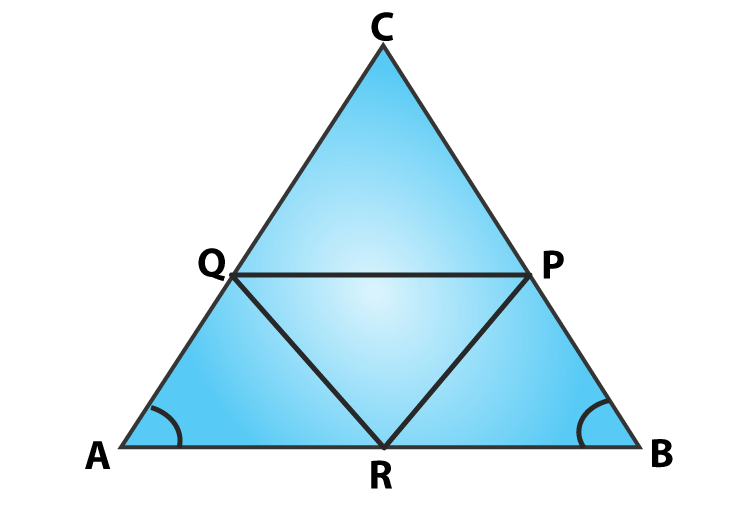In ΔABC,

R and P are mid points of AB and BC

By Mid-point Theorem

RP ∥ AC ⇒ RP = AC/2

RP ∥ AQ ⇒ RP = AQ

[A pair of side is parallel and equal]

Therefore, ARPQ is a parallelogram.

Now, AR = AB/2 = 30/2 = 15 cm

[AB = 30 cm (Given)]

AR = QP = 15 cm

[ Opposite sides are equal ]

And RP = AC/2 = 21/2 = 10.5 cm

[AC = 21 cm (Given)]

RP = AQ = 10.5cm

[ Opposite sides are equal ]

Now,

Perimeter of ARPQ = AR + QP + RP +AQ

= 15 +15 +10.5 +10.5

= 51

Perimeter of quadrilateral ARPQ is 51 cm.

Question 4: In a ΔABC median AD is produced to X such that AD = DX. Prove that ABXC is a parallelogram.

Solution: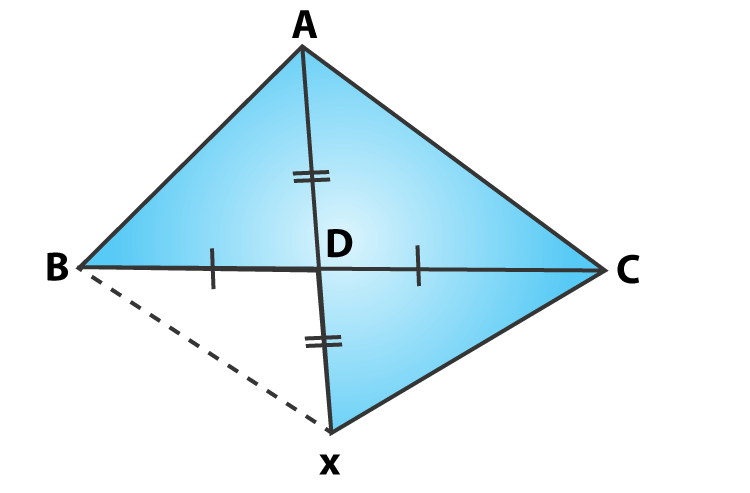BD = DC [Given]

From figure, Diagonals AX and BC bisect each other.

ABXC is a parallelogram.

Hence Proved.

Question 5: In a ΔABC, E and F are the mid-points of AC and AB respectively. The altitude AP to BC intersects FE at Q. Prove that AQ = QP.

Solution: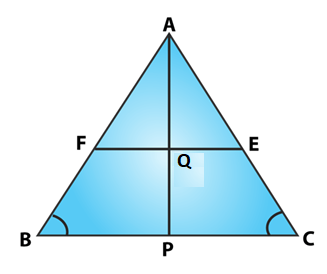In a ΔABC

E and F are mid points of AC and AB (Given)

EF ∥ BC ⇒ EF = BC/2 and

[By mid-point theorem]

In ΔABP

F is the mid-point of AB, again by mid-point theorem

FQ ∥ BP

Q is the mid-point of AP

AQ = QP

Hence Proved.

Question 6: In a ΔABC, BM and CN are perpendiculars from B and C respectively on any line passing through A. If L is the mid-point of BC, prove that ML = NL.

Solution: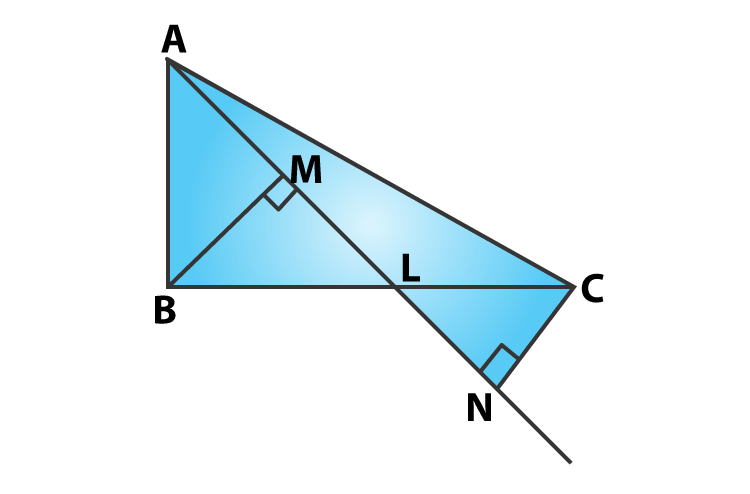Given that,

In ΔBLM and ΔCLN

∠BML = ∠CNL = 900

BL = CL [L is the mid-point of BC]

∠MLB = ∠NLC [Vertically opposite angle]

By ASA criterion:

ΔBLM ≅ ΔCLN

So, LM = LN [By CPCT]

Question 7: In figure, triangle ABC is a right-angled triangle at B. Given that AB = 9 cm, AC = 15 cm and D, E are the mid-points of the sides AB and AC respectively, calculate

(i) The length of BC (ii) The area of ΔADE.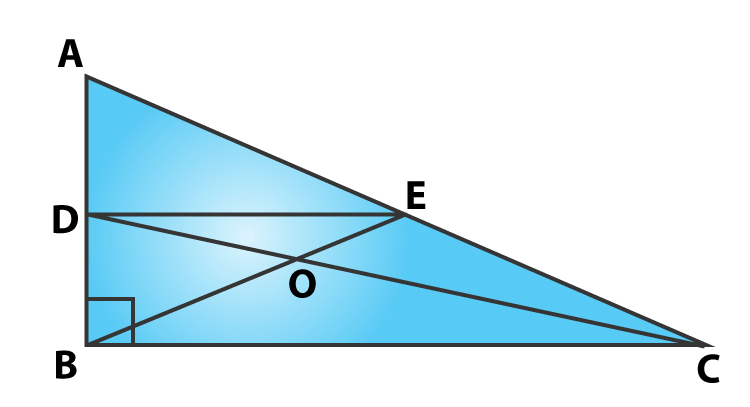Solution:

In ΔABC,

∠B=900 (Given)

AB = 9 cm, AC = 15 cm (Given)

By using Pythagoras theorem

AC2 = AB2 + BC2

⇒152 = 92 + BC2

⇒BC2 = 225 – 81 = 144

or BC = 12

Again,

AD = DB = AB/2 = 9/2 = 4.5 cm [D is the mid−point of AB

D and E are mid-points of AB and AC

DE ∥ BC ⇒ DE = BC/2 [By mid−point theorem]

Now,

= 1/2 x 4.5 x 6

=13.5

Area of ΔADE is 13.5 cm2

Question 8: In figure, M, N and P are mid-points of AB, AC and BC respectively. If MN = 3 cm, NP = 3.5 cm and MP = 2.5 cm, calculate BC, AB and AC.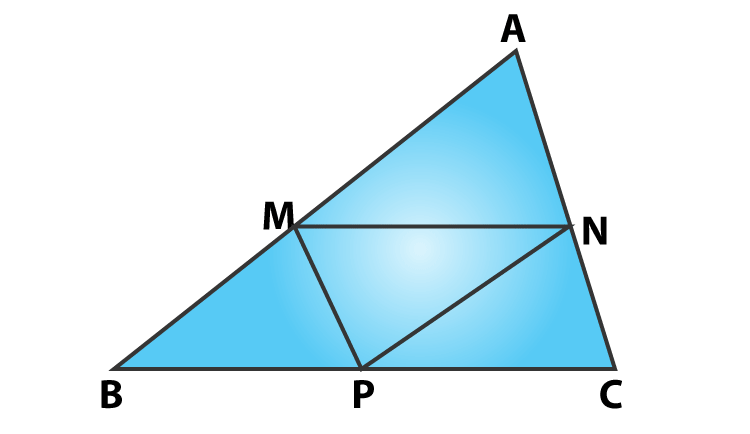Solution:

Given: MN = 3 cm, NP = 3.5 cm and MP = 2.5 cm.

M and N are mid-points of AB and AC

By mid−point theorem, we have

MN∥BC ⇒ MN = BC/2

or BC = 2MN

BC = 6 cm

[MN = 3 cm given)

Similarly,

AC = 2MP = 2 (2.5) = 5 cm

AB = 2 NP = 2 (3.5) = 7 cm

Question 9: ABC is a triangle and through A, B, C lines are drawn parallel to BC, CA and AB respectively intersecting at P, Q and R. Prove that the perimeter of ΔPQR is double the perimeter of ΔABC.

Solution: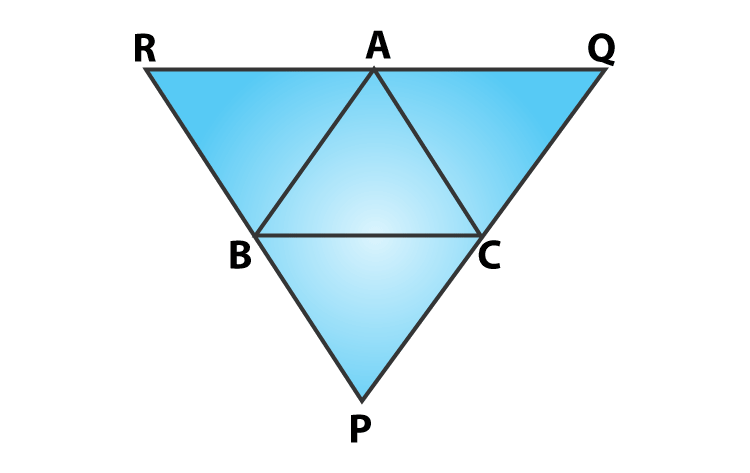ABCQ and ARBC are parallelograms.

Therefore, BC = AQ and BC = AR

⇒AQ = AR

⇒A is the mid-point of QR

Similarly B and C are the mid points of PR and PQ respectively.

By mid−point theorem, we have

AB = PQ/2, BC = QR/2 and CA = PR/2

or PQ = 2AB, QR = 2BC and PR = 2CA

⇒PQ + QR + RP = 2 (AB + BC + CA)

⇒ Perimeter of ΔPQR = 2 (Perimeter of ΔABC)

Hence proved.

Question 10: In figure, BE ⊥ AC, AD is any line from A to BC intersecting BE in H. P, Q and R are respectively the mid-points of AH, AB and BC. Prove that ∠PQR = 900.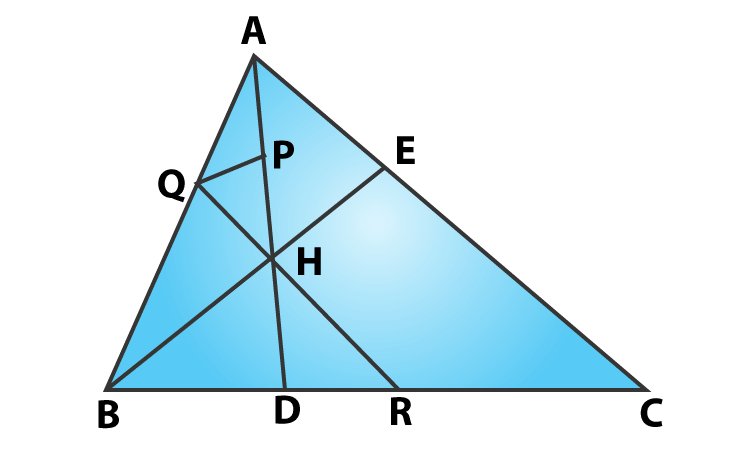Solution:

BE⊥AC and P, Q and R are respectively mid-point of AH, AB and BC. (Given)

In ΔABC, Q and R are mid-points of AB and BC respectively.

By Mid-point theorem:

QR ∥ AC …..(i)

In ΔABH, Q and P are the mid-points of AB and AH respectively

QP ∥ BH ….(ii)

But, BE⊥AC

From (i) and (ii) we have,

QP⊥QR

⇒∠PQR = 900

Hence Proved.

### Exercise VSAQs

Question 1: In a parallelogram ABCD, write the sum of angles A and B.

Solution:

In parallelogram ABCD, Adjacent angles of a parallelogram are supplementary.

Therefore, ∠A + ∠B = 1800

Question 2: In a parallelogram ABCD, if ∠D = 1150, then write the measure of ∠A.

Solution:

In a parallelogram ABCD,

∠D = 1150 (Given)

Since, ∠A and ∠D are adjacent angles of parallelogram.

We know, Adjacent angles of a parallelogram are supplementary.

∠A + ∠D = 1800

∠A = 1800 – 1150 = 650

Measure of ∠A is 650.

Question 3: PQRS is a square such that PR and SQ intersect at O. State the measure of ∠POQ.

Solution:

PQRS is a square such that PR and SQ intersect at O. (Given)

We know, diagonals of a square bisects each other at 90 degrees.

So, ∠POQ = 900

Question 4: In a quadrilateral ABCD, bisectors of angles A and B intersect at O such that ∠AOB = 75°, then write the value of ∠C + ∠D.

Solution:

∠AOB = 75o (given)

In a quadrilateral ABCD, bisectors of angles A and B intersect at O, then

∠AOB = 1/2 (∠ADC + ∠ABC)

or ∠AOB = 1/2 (∠D + ∠C)

By substituting given values, we get

75 o = 1/2 (∠D + ∠C)

or ∠C + ∠D = 150 o

Question 5: The diagonals of a rectangle ABCD meet at O. If ∠BOC = 44o, find ∠OAD.

Solution:

ABCD is a rectangle and ∠BOC = 44o (given)

∠AOD = ∠BOC (vertically opposite angles)

∠AOD = ∠BOC = 44o

∠OAD = ∠ODA (Angles facing same side)

and OD = OA

Since sum of all the angles of a triangle is 180 o, then

So, ∠OAD = 1/2 (180 o – 44 o) = 68 o

Question 6: If PQRS is a square, then write the measure of ∠SRP.

Solution:

PQRS is a square.

⇒ All side are equal, and each angle is 90o degrees and diagonals bisect the angles.

So, ∠SRP = 1/2 (90 o) = 45o

Question 7: If ABCD is a rectangle with ∠BAC = 32o, find the measure of ∠DBC.

Solution:

ABCD is a rectangle and ∠BAC=32 o (given)

We know, diagonals of a rectangle bisects each other.

AO = BO

∠DBA = ∠BAC = 32 o (Angles facing same side)

Each angle of a rectangle = 90 degrees

So, ∠DBC + ∠DBA = 90 o

or ∠DBC + 32 o = 90 o

or ∠DBC = 58 o

Question 8: If ABCD is a rhombus with ∠ABC = 56o, find the measure of ∠ACD.

Solution:

In a rhombus ABCD,

<ABC = 56 o

So, <BCD = 2 (<ACD) (Diagonals of a rhombus bisect the interior angles)

or <ACD = 1/2 (<BCD) …..(1)

We know, consecutive angles of a rhombus are supplementary.

∠BCD + ∠ABC = 180 o

∠BCD = 180 o – 56 o = 124 o

Equation (1) ⇒ <ACD = 1/2 x 124 o = 62 o

Question 9: The perimeter of a parallelogram is 22 cm. If the longer side measure 6.5 cm, what is the measure of shorter side?

Solution:

Perimeter of a parallelogram = 22 cm. (Given)

Longer side = 6.5 cm

Let x be the shorter side.

Perimeter = 2x + 2×6.5

22 = 2x + 13

2x = 22 – 13 = 9

or x = 4.5

Measure of shorter side is 4.5 cm.

Question 10: If the angles of a quadrilateral are in the ratio 3:5:9:13, then find the measure of the smallest angle.

Solution:

Angles of a quadrilateral are in the ratio 3 : 5 : 9 : 13 (Given)

Let the sides are 3x, 5x, 9x, 13x

We know, sum of all the angles of a quadrilateral = 360o

3x + 5x + 9x + 13x = 360 o

30 x = 360 o

x = 12 o

Measure of smallest angle = 3x = 3(12) = 36o .

## RD Sharma Solutions for Class 9 Maths Chapter 14 Quadrilaterals

In the 14th Chapter of Class 9, RD Sharma Solutions students will study important concepts. Some are listed below:

• Angle sum property.
• Conditions for Quadrilaterals to be a Parallelogram.

## Frequently Asked Questions on RD Sharma Solutions for Class 9 Maths Chapter 14

### What are the key benefits of RD Sharma Solutions for Class 9 Maths Chapter 14?

RD Sharma Solutions Class 9 Chapter 14 provides the textbook questions in a more precise manner for better learning of concepts. The solutions are well structured by subject experts after conducting vast research on each concept. Students can make use of these solutions in PDF format for thorough exam preparation whenever required.

### How RD Sharma Solutions for Class 9 Maths Chapter 14 is helpful to score high marks among CBSE students?

Following RD Sharma Solutions helps students to clear their doubts quickly which arise while revising the textbook problems. Continuous practice of these solutions enhances the skills which are vital from an exam perspective. The solutions are developed in a comprehensive manner by expert faculty to help CBSE students to achieve their desired scores in exams.

### Is it important to practice RD Sharma Solutions for Class 9 Maths Chapter 14 on a daily basis?

Yes, students who practice RD Sharma Solutions Class 9 Chapter 14 on a daily basis understand the complex concepts in an easy manner. These solutions provide a clear explanation for each step which in turn helps students to grasp various methods of solving the problems with ease. Expert faculty designed the solutions in an accurate manner with the intention to boost conceptual knowledge among students.